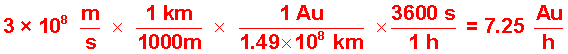Section 7 & 9

Quiz ( 1)-Ch(1)

Key                                 Phys 101 ( Term 032)-( F. Enaya)** Show your steps clearly for full credit.!! **

Q1. The acceleration  { a } of an object moving along x-axis is given by:       a = x n  v p  m q

where { x } is the distance, { v } is the speed and { m } is the mass of the object. Find the values of  {n}, {p} and {q} so that the above equation is dimensionally correct.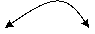Using basic Units ( L, T and M)  :   a = x n  v p  m q Þ (L/T 2) = Ln . (L/T) p . M q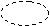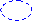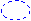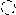Þ     L1 . T-2 = L ( n + p) . T p . M q    Þ

n + p = 1   , and   - p = -2  Þ     ( p = 2)  & ( n = - 1), where there is no (M) in the left side Þ     (q = 0)

Q2. An astronomical unit (AU), is the average distance of the earth from the sun, approximately 1.49´108 km.

The speed of the light is about  3´108 m/s. Express the speed of light in terms of astronomical units per hour.